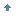# 诊断 Java 代码: 使用静态类型的理由

• 通过早期错误检测，提高健壮性
• 通过在最佳的时候作所需的检查，提高性能
• 弥补单元测试的缺点

Sun JSR14 编译器在语言中增加了形式有限的泛型（也称为 参数化） 类型；我们相信它迟早会被加入该语言，因为它目前在 Java 社区过程中得到了坚定的支持。更高级的语言扩展（例如 NextGen）承诺在 JSR14 提供的增加的表达力上更上一层楼。那是好事，因为在很多环境下 NextGen 都有助于减少甚至在 JSR14 中也是需要的一些增加的复杂性。请参阅 参考资料找到关于这个问题的更多信息。（除了 JSR14 链接，还有些关于 参数的多态性的文章。）NextGen 之路 在 Rice University，一个编程语言技术组实现了 NextGen，它是向上兼容版的 GJ 编译器。 GJ 是由 Martin Odersky 写的编译器，它允许用户在 Java 语言中增加 泛型，也称为 参数化类型而不牺牲与旧代码的兼容性。它的前身被称为 Pizza；是一个实验性的语言，它的一些功能被 Java 1.2 采纳。GJ 对 Pizza 的改进仅在于填加了泛型类型。 GJ 把源代码编译成字节码是通过 类型消除（type erasure），它是把每个类型的变量的每个实例替换成该变量上限的方法。GJ 还允许将类型变量声明为只用于某些特定方法，而不是用于整个类。 NextGen 语言由 Rice University 的 Robert Cartwright 和 Sun Microsystems 的 Guy Steele 共同开发。该语言在 GJ 中加入了进行类型变量的运行时检查的能力。它支持个别方法的类型参数化，支持内部类和泛型类型的运行时运算。它不允许用基本类型初始化类型变量。回页首回页首

 `import junit.framework.*;import java.io.*;/** * A test class for MultiSet. * */public class MultiSetTest extends TestCase { private static String W = "w"; private static String X = "x"; private static String Y = "y"; private static String Z = "z"; private static MultiSet EMPTY = new MultiSet(); private static MultiSet XY = new MultiSet(X, Y); private static MultiSet YZ = new MultiSet(Y, Z); private static MultiSet XYZ = new MultiSet(X, Y, Z); private static MultiSet XYY = new MultiSet(X, Y, Y); private static MultiSet WXY = new MultiSet(W, X, Y); /** * Constructor. * @param String name */ public MultiSetTest(String name) { super(name); } /** * Creates a test suite for JUnit to run. * @return a test suite based on the methods in this class */ public static Test suite() { return new TestSuite(MultiSetTest.class); } private void _assertOrder(MultiSet set, String key, int value) { assertEquals("order for key " + key, value, set.order(key)); } public void testEmpty() { _assertOrder(EMPTY, X, 0); _assertOrder(EMPTY, Y, 0); _assertOrder(EMPTY, Z, 0); } public void testOrder() { _assertOrder(XY, X, 1); _assertOrder(XY, Y, 1); _assertOrder(YZ, Y, 1); _assertOrder(YZ, Z, 1); } public void testAdd() { MultiSet added = XY.add(YZ); _assertOrder(added, X, 1); _assertOrder(added, Y, 2); _assertOrder(added, Z, 1); } public void testSubset() { assertTrue(XY.subset(XYZ)); assertTrue(YZ.subset(XYZ)); assertTrue(! YZ.subset(XY)); assertTrue(! XY.subset(YZ)); assertTrue(! XYZ.subset(XY)); assertTrue(! XYZ.subset(YZ)); assertTrue(! XYY.subset(XYZ)); assertTrue(! XYZ.subset(XYY)); } public void testSubtract() { MultiSet XYYZ = XY.add(YZ); assertEquals(YZ, XYYZ.subtract(WXY)); assertEquals(YZ, XYYZ.subtract(XY)); assertEquals(XY, XYYZ.subtract(YZ)); assertEquals(EMPTY, EMPTY.subtract(YZ)); assertEquals(EMPTY, YZ.subtract(YZ)); } public void testUnion() { assertEquals(XYZ, XY.union(YZ)); } public void testIsEmpty() { assertTrue(EMPTY.isEmpty()); assertTrue(! XY.isEmpty()); }}`回页首回页首

• 博文量
1714
• 访问量
1291044